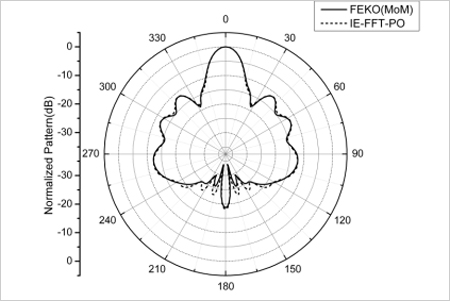# Efficient IE-FFT and PO Hybrid Analysis of Antennas around Electrically Large Platforms_jinlong

42
Issuing time:2016-05-27 23:13Author:Ji Ma

Abstract: An  efficient  hybrid  analysis  which  combines  integral  equations  (IE)  with physical optics (PO) approximation is presented for antennas around an electrically large platform. In the analysis, the whole surface of the platform and antennas is divided into two regions, namely, the full-wave region and the PO region. In the full-wave region, the IE  is  modified  by coupling  into  the  PO  contribution.  Similar  to  the  fast  IE  method,  the modified  impedance  matrix  is  decomposed  into  the  near  field  couplings  and  the well-separated group couplings, respectively. By interpolating the Green’s function, the couplings  between  two  well-separated  groups  can  be  computed  using  fast  Fourier transform (FFT). Due to the PO approximation, the hybrid method utilizes fewer unknowns and  requires  less  solution  time  than  the  conventional  IE-FFT.  Finally,  the  proposed method has proved to be valid by comparing with the EM software FEKO.The  method  of  moments  (MoM)  has  been  very  popular  in  solving  the electromagnetic problems of 3-D structures. However, in the situation that antennas are located near an electrically large platform, the efficiency of the conventional MoM may be lost due to excessive computer requirement. Hybrid method which combines MoM with  physical  optics  (PO)  asymptotic  method  appears  to  be  able  to  well  handle  such problems.  In  this  current-based  hybrid  analysis,  the  impedance  matrix  is  modified  by coupling  into  the  PO  contribution.  Each  MoM  basis  function  interacts  with  other  MoM basis functions directly and through each PO triangle indirectly. Therefore, it is necessary to have as small a MoM region as possible. Otherwise, calculating the interaction between the  MoM  and  PO  regions  will  lead  much  longer  solution  time,  which  may  make  the analysis of electrically large problems become prohibitive.

Another efficient analysis of the problems can be fast integral equation (IE) methods. Recent  advancements  of fast multipole method  (FMM)  and  its  extension  called multilevel fast multipole algorithm (MLFMA) have made significant inroads toward solving realistic large-size problems. However, both the methods strongly depend on the integral kernels. The major drawback makes the application of FMM and MLFMA confined. On the other hand, there is another class of fast IE methods called grid-based approaches, such as precorrected-FFT (pFFT), the adaptive integral method (AIM) , and the algorithm of sparse-matrix/canonical-grid (SM/CG). In 2005, the IE-FFT algorithm was proposed by Seung Mo Seo and Jin-Fa Lee. This method interpolates the Green’s function  on  a  regular  Cartesian  grid  and  is  easier  to  implement  than  other  grid-based approaches. All of these fast IE methods require less storage and lower complexity than the conventional MoM.

This paper presents a novel hybrid technique, which combines the IE-FFT method with PO method. The surfaces of the platform and antennas are modeled by triangular meshes. Antennas are assigned to be the full-wave region and the platform is chosen as the PO region. Thus the electric field integral equation (EFIE) in which the electric field radiated by the PO current is taken into account can be obtained in the full-wave region. Similar to the conventional IE-FFT, the modified impedance matrix is decomposed into the near field couplings and the well-separated group couplings, respectively. As a result of the Green’s function interpolated  on  a  regular  Cartesian  grid,  the  couplings  between  two well-separated  groups  can  be  computed  using  FFT. Compared  with  the  conventional IE-FFT, the proposed technique needs fewer unknowns and requires less solution time. On the other hand, the method has proved to be valid by comparing with the EM software FEKO.